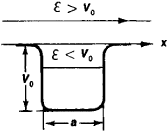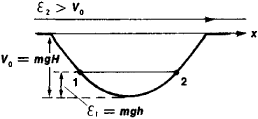# Potential Well

(redirected from Quantum confinement)
Also found in: Dictionary.
Related to Quantum confinement: Quantum confinement effect

## potential well

[pə′ten·chəl ¦wel]
(physics)
For an object in a conservative field of force, a region in which the object has a lower potential energy than in all the surrounding regions.

## Potential Well

in physics, a bounded region of space in which the potential energy of a particle is less than that outside the region. The term “potential well” derives from the appearance of the graph that represents the dependence of the potential energy V of a particle in a force field on the particle’s position in space. (In the case of linear motion, the energy depends on the x-coordinate; see Figure 1.) This form of the function V(x) arises in a field of attractive forces. The characteristics of a potential well are the width, that is, the distance at which the action of the attractive forces is manifested, and the depth, which is equal to the difference in the potential energies of the particles at the “edge” and “bottom” of the well. The bottom corresponds to the minimum potential energy. The main property of a potential well is its ability to confine a particle whose total energy ℰ is less than the depth of the well V0; such a particle within a potential well will be in a bound state.Figure 1. Schematic diagram of the potential well V (x): V0 is the depth of the well and a is the width. The total energy £ of a particle is conserved and therefore is represented on the graph by a horizontal line.

In classical mechanics, a particle with energy ℰ < Vo will be unable to escape from the potential well and will always move in the bounded region of the well. The particle’s position at the bottom of the well corresponds to a stable equilibrium and is reached when the particle’s kinetic energy ℰ kin = &— V = 0. If ℰ > Vo, then the particle will overcome the effect of the attractive forces and escape from the well. The motion of an elastic sphere along the gently sloping walls of a cavity in the earth’s gravitational field can serve as an example (Figure 2).Figure 2. A sphere of mass m with energy ℇ1 < V0 cannot escape from the cavity. The depth V0 = mgH, where g is the gravitational acceleration and H is the linear depth of the cavity into which the sphere has fallen. If friction is disregarded, the sphere will oscillate between points 1 and 2, rising only to the height h =1/mg. If the energy of the sphere is ℇ1 > V0, it will escape from the cavity and move toward infinity with a constant velocity ν determined by the relation mv2/2 =2 V0.

In quantum mechanics, in contrast to classical mechanics, the energy of a particle in a bound state in a potential well cai. assume only certain discrete values; that is, there exist discrete energy levels. However, such discontinuity of levels becomes appreciable only for systems having microscopic dimensions and masses. The interval Δ ℰ between energy levels for a particle of mass m in a “deep” well of width a is of the order of the magnitude Δ ℰ ≃ ℏ2/ma2, where ℏ is Planck’s constant. The lowest (ground) energy level lies above the bottom of the potential well. In a well of small depth, that is, V0 ≤ ℏ, a bound state may be absent altogether. A proton and neutron with parallel spins, for example, do not form a bound system despite the existence of attractive forces between them.

Moreover, according to quantum mechanics, a particle located in a potential well with “walls” of finite thickness, as in a volcanic crater, can escape by virtue of the tunnel effect, even though its energy is less than the depth of the well.

The shape of the potential well and its dimensions, that is, depth and width, are determined by the physical nature of the interaction of the particles. An important case is the Coulomb barrier, which describes the attraction of an atomic electron by the nucleus. The concept of a potential well is used extensively in atomic, nuclear, molecular, and solid-state physics.

References in periodicals archive ?
conclude that the quantum confinement effect dominates the electronic and optical properties of the NBE-T-CQDs.
Quantum Confinement. Many of the trends observed experimentally for the UV-Vis-near-IR optical response of Bi nanostructures can be qualitatively accounted for by classical modeling.
This interval was chosen to examine the typical electronic transition bands associated with the quantum confinement effect.
This part begins by first describing the quantum confinement model in the case of silicon; then proceeds to the preparation strategies where the importance of surface chemistry will be highlighted; this section completes by covering the use of silicon quantum dots for biosensing and imaging applications.
When it is assumed that the threshold voltage rising stems from the quantum confinement [3, 6, 15], [V.sub.th] for ultrathin-body n-channel SOI MOSFET can be expressed as 
The size dependence of band gap of the nanoparticles according to quantum confinement model is expected to be of the form:
The quantum confinement effect is maximum in the less than 100greater than wire which causes higher band gap and heavier effective mass for reasonably smaller diameter wires.
It has been established that the spontaneous polarization, valence band offset, and quantum confinement effect were three key effects in the heterocrystalline superlattice which should be considered .
Due to the quantum confinement effect, the optical property and the band gap of a QD can be adjusted by changing the size of the QDs [2-4].
The [delta]-doping technique has been used to provide the carrier quantum confinement effects.
This work considers a new paradigm in photovoltaic conversion, using quantum confinement as well as light and thermal management.

Site: Follow: Share:
Open / Close